• +91 9971497814
• info@interviewmaterial.com

# RD Chapter 3- Pair of Linear Equations in Two Variables Ex-3.6 Interview Questions Answers

### Related Subjects

Question 1 : 5 pens and 6 pencils together cost ₹ 9 and 3 pens and 2 pencils cost ₹ 5. Find the cost of 1 pen and 1 pencil. (C.B.S.E. 1991)

Let cost of 1 pen = ₹ x
and cost of 1 pencil = ₹ y
According to the conditions,
5x + 6y = 9 ….(i)
3x + 2y = 5 …(ii)
Multiplying (i) by 1 and (ii) by 3, we get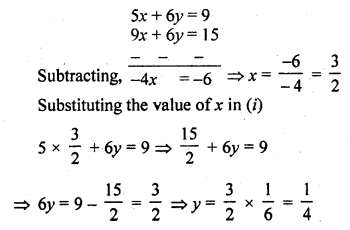Cost of one pen = ₹ 32
and cost of one pencil = ₹ 14

Question 2 : 7 audio cassettes and 3 video cassettes cost ₹ 1110, while 5 audio cassettes and 4 video cassettes cost ₹ 1350. Find the cost of an audio cassette and a video cassette. (C.B.S.E. 1992)

Let the cost of 1 audio cassette = ₹ x
and cost of 1 video cassette = ₹ y
According to the condition,
7x + 3y= 1110 ….(i)
5x + 4y = 1350 ….(ii)
Multiplying (i) by 4 and (ii) by 3,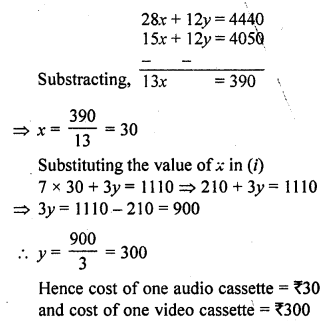Question 3 : Reena has pens and pencils which together are 40 in number. If she has 5 more pencils and 5 less pens, then number of pencils would become 4 times the number of pens. Find,.the original number of pens and pencils. (C.B.S.E 1992C)

Let number of pens = x
and number of pencils = y
x + y = 40 ….(i)
In second case,
number of pens = x – 5
and number of pencils = y + 5
(y + 5) = 4 (x – 5) => y + 5 = 4x – 20
4x – y = 5 + 20 => 4x – y = 25 ….(ii)
5x = 65 => x = 13 [From (i) ]
13 + y = 40 => y = 40 – 13 = 27
Hence number of pens = 13
and number of pencils = 27

Question 4 : 4 tables and 3 chairs, together, cost ₹ 2,250 and 3 tables and 4 chairs cost ₹ 1950. Find the cost of 2 chairs and 1 table.

Let cost of 1 table = ₹ x
and cost of 1 chair = ₹ y
According to the conditions,
4x + 3y = 2250 ….(i)
3x + 4y= 1950 ….(ii)
Multiplying (i) by 4 and (ii) by 3, we get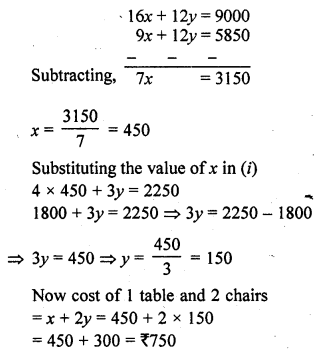Question 5 : 3 bags and 4 pens together cost ₹ 257 whereas 4 bags and 3 pens together cost ₹ 324. Find the total cost of 1 bag and 10 pens. (C.B.S.E. 1996)

Let cost of 1 bag = ₹ x
and cost of 1 pen = ₹ y
According to the conditions,
3x + 4y = 257 ….(i)
4x + 3y = 324 ….(ii)
Multiplying (i) by 3 and (ii) by 4, we get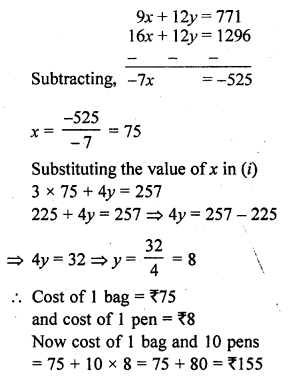Question 6 : 5 books and 7 pens together cost ₹ 79 whereas 7 books and 5 pens together cost ₹ 77. Find the cost of 1 book and 2 pens. (C.B.S.E. 1996)

Let the cost 1 book = ₹ x
and cost of 1 pen = ₹ y
Now according to the conditions,
5x + 7y = 79 ….(i)
7x + 5y = 77 ….(ii)
Multiplying (i) by 5 and (ii) by 7, we get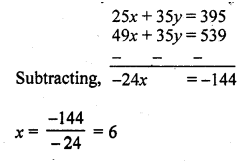Substituting the value of x in (i)
5 x 6 + 7y = 79
=> 30 + 7y = 79
=> 7y = 79 – 30 = 49
y = 7
Cost of 1 book and 2 pens = 6 + 2 x 7 = 6 + 14 = 20

Question 7 : Jamila sold a table and a chair for ₹ 1050, thereby making a profit of 10% on a table and 25% on the chair. If she had taken profit of 25% on the table and 10% on the chair she would have got ₹ 1065. Find the cost price of each. [NCERT Exemplar]

Let the cost price of the table be ₹ x
and the cost price of the chair by ₹ y.
The selling price of the table, when it is sold at a profit of 10%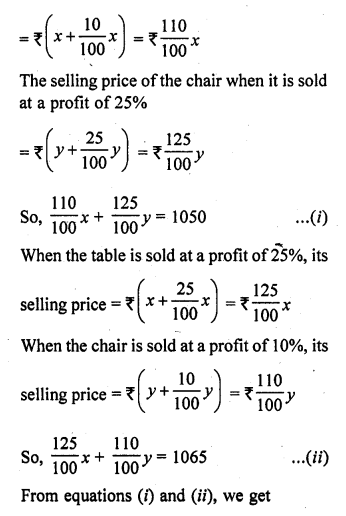110x + 125y = 105000
and 125x + 110y = 106500
On adding and subtracting these equations, we get
235x + 235y = 211500
and 15x – 15y= 1500
i.e., x + y = 900 …(iii)
and x – y = 100 …(iv)
Solving equation (iii) and (iv), we get
2x = 1000
x = 500
500 + y = 900
=> y = 900 – 500
y = 400
x = 500, y = 400
So, the cost price of the table is ₹ 500 and the cost price of the chair is ₹ 400.

Question 8 :
Susan invested certain amount of money in two schemes A and B, which offer interest at the rate of 8% per annum and 9% per annum, respectively. She received ₹ 1860 as annual interest. However, had she interchanged the amount of investment in the two schemes, she would have received 720 more as annual interest. How much money did she invest in each scheme?
[NCERT Exemplar]

Let the amount of investments in schemes A and B be ₹ x and ₹ y, respectively.
Case I:
Interest at the rate of 8% per annum on scheme A + Interest at the rate of 9% per annum on scheme B = Total amount received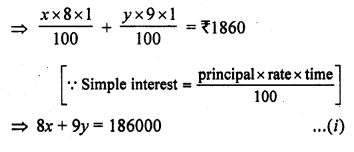Case II:
Interest at the rate of 9% per annum on scheme A + Interest at the rate of 8% per annum on scheme B = ₹ 20 more as annual interest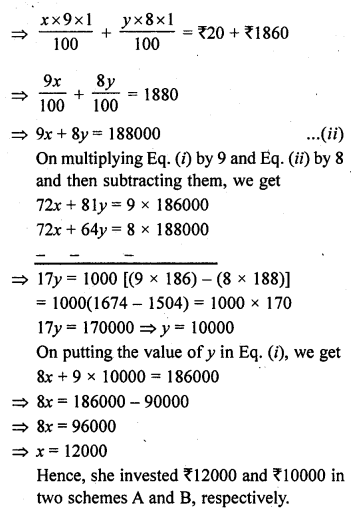Question 9 : The coach of a cricket team buys 7 bats and 6 balls for ₹ 3800. Later, he buys 3 bats and 5 balls for ₹ 1750. Find the cost of each bat and each ball.

Let cost of 1 bat = ₹ x
and cost of 1 ball = ₹ y
According to the conditions,
7x + 6y = 3800 ….(i)
3x + 5y = 1750 ….(ii)
Multiplying (i) by 5 and (ii) by 6, we get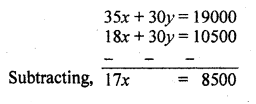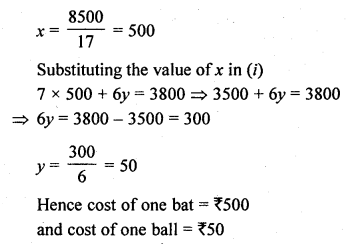Question 10 : A lending library has a fixed charge for the first three days and an additional charge for each day thereafter. Saritha paid ₹ 27 for a book kept for seven days, while Susy paid ₹ 21 for the book she kept for five days. Find the fixed charge and the charge for each extra day;

Let the fixed charge for the book = ₹ x
and let extra charge for each day = ₹ y
According to the given conditions,
x + 4y = 27 ….(i)
x + 2y = 21 ….(ii)
Subtracting,
2y = 6 => y = 3
Substituting the value of y in (i)
x + 4 x 3 = 27
=> x + 12 = 27
=> x = 27 – 12 = 15
Amount of fixed charge = ₹ 15
and charges for each extra day = ₹ 3

Todays Deals### RD Chapter 3- Pair of Linear Equations in Two Variables Ex-3.6 Contributorskrishan

Name:
Email:

# Latest News# 9000 interview questions in different categories